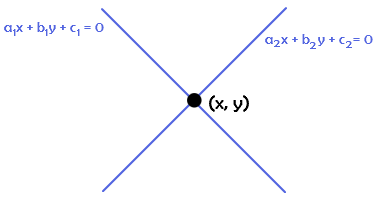# Point of Intersection Formula

Point of intersection means the point at which two lines intersect. These two lines are represented by the equation a1x+ b1x + c1= 0  and a2x+ b2x + c2 = 0 respectively. Given figure illustrate the point of intersection of two lines.We can find the point of intersection of three or more lines also. By solving the two equations, we can find the solution for point of intersection of two lines.### Solved Examples

Question 1: Find out the point of intersection of two lines $x^{2}$ + 2x + 1 = 0 and 2$x^{2}$ + 3x + 5 = 0 ?

Solution:

Given straight line equations are: $x^{2}$ + 2x + 1 = 0 and 2$x^{2}$ + 3x + 5 = 0

Here,
a1 = 1
b1 = 2
c1 = 1

a2 = 2, b2 = 3, c2 = 5

Intersection point can be calculated using this formula,

x = $\frac{b_{1}c_{2}-b_{2}c_{1}}{a_{1}b_{2}-a_{2}b_{1}}$; y = $\frac{a_{2}c_{1}-a_{1}c_{2}}{a_{1}b_{2}-a_{2}b_{1}}$

(x,y) = ($\frac{2\times5-3\times1}{1\times3-2\times2}$,$\frac{2\times1-1\times5}{1\times3-2\times2}$)

(x,y) = ($\frac{10-3}{3-4}$,$\frac{2-5}{3-4}$)

(x,y) = (-7,3)